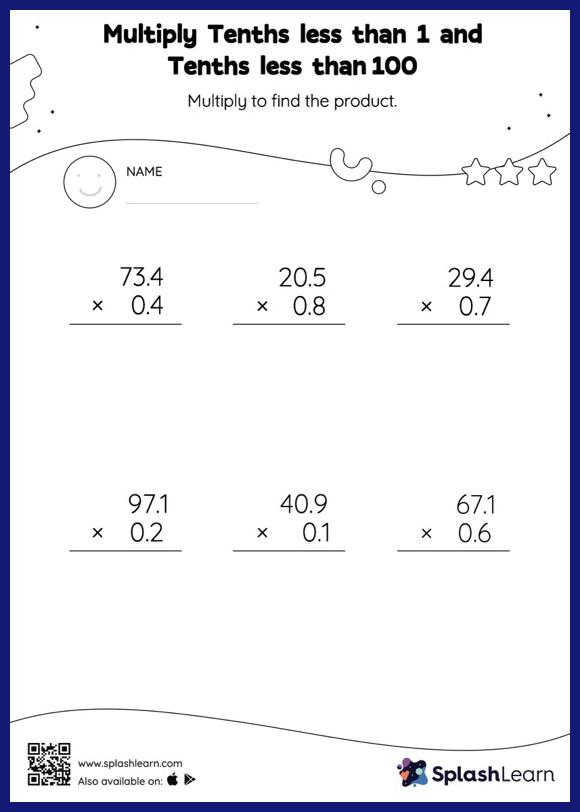# Multiply Tenths less than 1 and Tenths less than 100: Vertical Multiplication Worksheet

Home > Multiply Tenths less than 1 and Tenths less than 100: Vertical MultiplicationThis worksheet invites students to multiply tenths less than 1 and tenths less than 100, which helps them become proficient in multiplication. When a tenth is multiplied by another tenth, the product will have two decimal digits. Students first multiply the numbers ignoring the decimal points, and then add the decimal point two places from the right in the product. They practice this concept extensively in multiply tenths less than 1 and tenths less than 100 worksheet. In this worksheet, numbers are laid one on top of another (vertical format). This encourages students to use the inherent place value structure to solve the problems.